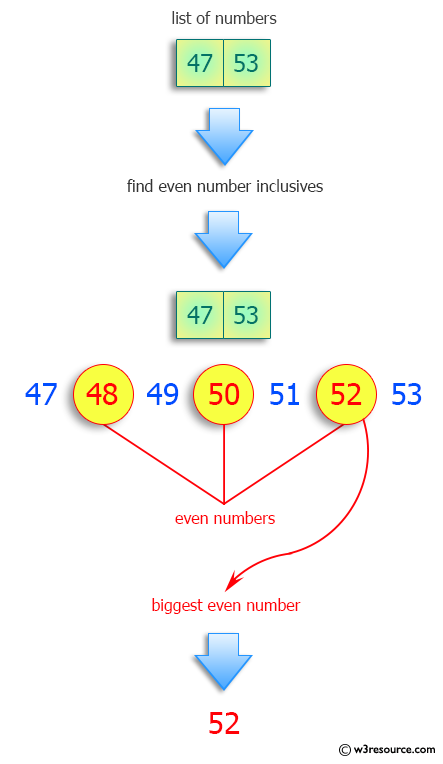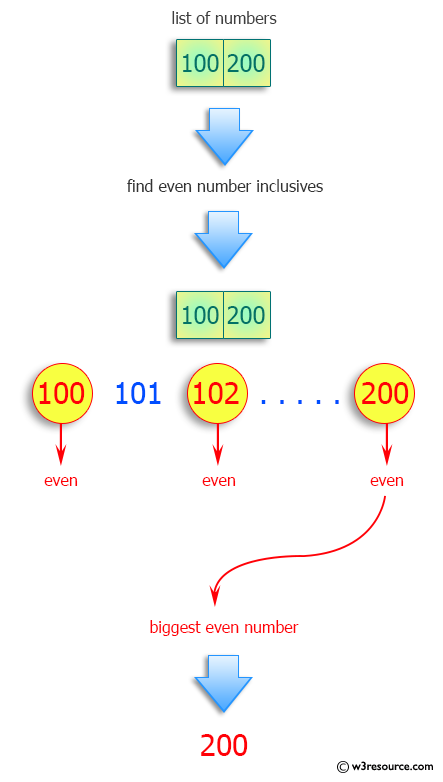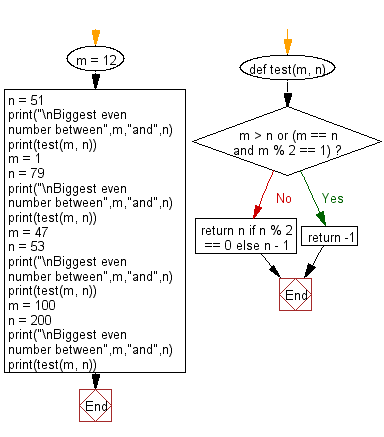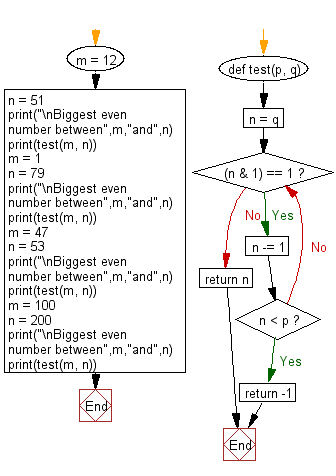﻿ Python: Biggest even number between two numbers inclusive - w3resource# Python: Biggest even number between two numbers inclusive

## Python Programming Puzzles: Exercise-58 with Solution

Write a Python program to find the biggest even number between two numbers inclusive.

```Input:
m = 12
n = 51
Output:
50
Input:
m = 1
n = 79
Output:
78
Input:
m = 47
n = 53
Output:
52
Input:
m = 100
n = 200
Output:
200
```

Pictorial Presentation:Sample Solution-1:

Python Code:

``````def test(m, n):
if m > n or (m == n and m % 2 == 1):
return -1
return n if n % 2 == 0 else n - 1

m = 12
n = 51
print("\nBiggest even number between",m,"and",n)
print(test(m, n))
m = 1
n = 79
print("\nBiggest even number between",m,"and",n)
print(test(m, n))
m = 47
n = 53
print("\nBiggest even number between",m,"and",n)
print(test(m, n))
m = 100
n = 200
print("\nBiggest even number between",m,"and",n)
print(test(m, n))
``````

Sample Output:

```Biggest even number between 12 and 51
50

Biggest even number between 1 and 79
78

Biggest even number between 47 and 53
52

Biggest even number between 100 and 200
200
```

Flowchart:## Visualize Python code execution:

The following tool visualize what the computer is doing step-by-step as it executes the said program:

Sample Solution-2:

Python Code:

``````def test(p, q):
n = q
while (n & 1) == 1:
n -= 1
if n < p:
return -1
return n
m = 12
n = 51
print("\nBiggest even number between",m,"and",n)
print(test(m, n))
m = 1
n = 79
print("\nBiggest even number between",m,"and",n)
print(test(m, n))
m = 47
n = 53
print("\nBiggest even number between",m,"and",n)
print(test(m, n))
m = 100
n = 200
print("\nBiggest even number between",m,"and",n)
print(test(m, n))
``````

Sample Output:

```Biggest even number between 12 and 51
50

Biggest even number between 1 and 79
78

Biggest even number between 47 and 53
52

Biggest even number between 100 and 200
200
```

Flowchart:## Visualize Python code execution:

The following tool visualize what the computer is doing step-by-step as it executes the said program:

Python Code Editor :

Have another way to solve this solution? Contribute your code (and comments) through Disqus.

What is the difficulty level of this exercise?

Test your Programming skills with w3resource's quiz.

﻿

## Python: Tips of the Day

Clamps num within the inclusive range specified by the boundary values x and y:

Example:

```def tips_clamp_num(num,x,y):
return max(min(num, max(x, y)), min(x, y))
print(tips_clamp_num(2, 4, 6))
print(tips_clamp_num(1, -1, -6))
```

Output:

```4
-1
```